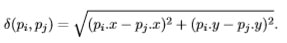```Coursework
University of Surrey
```

## 1 Time Complexity

[fundamental skills and numeracy] The purpose of this task is to strengthen your familiarity with growth rates of running times.

### 1.1 Limits on the input size

Suppose you have algorithms with the ﬁve running times listed below. (Assume these are the exact number of operations performed as a function of the input size n). Suppose your computer runs with 10 GHz, ie can perform 1010 operations per second, and you need to compute a result in at most one hour of computation. For each of the algorithms, what is the largest input n for which you would be able to get the result within an hour?

1. f(n) = n2
2. f(n) = n3
3. f(n) = 100n3
4. f(n) = nlog(n)
5. f(n) = 2n
6. Finally, what is the ratio between the largest and smallest number of inputs that can be processed within an hour for algorithms with the above running times?

### 1.2 Recurrence relations

For each of the following recurrence relations determine values a,b,c and possibly k to insert into the master theorem as presented in the lecture. Determine which case of the master theorem applies and give the solution for T(n) if the relation can be solved using one of the cases of the Master Theorem. Otherwise, explain why the Master Theorem is not applicable.

1. T(n) = 9T(n/9) + n2
2. T(n) = 9/4 T(2/3 n) + n
3. T(n) = 8T(n/4) + n3/2
4. T(n) = (4/3)n T (n/4) + n2
5. T(n) = √2T (n/2) + log n

## 2 2D Minimal Distance

This exercise is about applying one of the algorithms from the lecture on a small scale by hand to experience how a divide-and-conquer algorithm works. In your solution, refer to the points given by their label pi rather than just be their coordinates.

It is essential that you follow the algorithm.Any graphs or graphical argumentation you might ﬁnd helpful for your own understanding of the algorithm will be ignored for marking as they are not part of the algorithm.

Let the set S = {`pi|i = 1,2,··· ,8`} contain the following points:

```p1 = (10,9) p2 = (12,−11) p3 = (−11,8) p4 = (−6,−7)
p5 = (15,8) p6 = (14,−10) p7 = (−5,−4) p8 = (−9,−6)
```

Find the minimal distance of points within the set using the algorithm from the lecture. Calculate distances using the Euclidean metric### 3 Designing an algorithm

Consider the following basic problem: You are given an array A consisting of n integers A,A,··· ,A[n]. You would like to output a 2D n × n array B in which B[i,j] (for i < j) contains the sum of array entries from A[i] through A[j] – that is the sum A[i] + A[i + 1] +···A[j]. The entry B[i,i] shall have the value A[i] (ie the sum running from A[i] to A[i]). (The value of entries B[i,j] is left unspeciﬁed whenever i > j, so it does not matter what is output for these values, or whether there is a value at all.)

Here is a simple brute force algorithm to solve this problem:

1. for i = 1,2,···n do
2. for j = i + 1,i + 2,···n do
3. Add up entries A[i] to A[j].
4. Store the result in B[i,j].
5. end for
6. end for
7. Return B.

1. Give a big-O bound on the running time of this algorithms in terms of n and the reasoning which leads up to your result for the big-O.

2. Although the algorithm is the most straight forward way to solve the problem, it contains some unnecessary sources of ineﬃciency. Find a diﬀerent algorithm with a lower order of running time, and write it down in pseudo-code with a lower order of running time.